# Interpreting tandem mass spectra of peptides (Proteomics)

## 1. Introduction

Given the success of database search programs at identifying proteins (Clauser et al., 1999; Eng et al., 1994; Fenyo et al., 1998; Mann and Wilm, 1994; Perkins et al., 1999), one could reasonably question the importance of knowing how to manually interpret tandem mass spectra (MS/MS) of peptides. This is especially true when working in a high-throughput environment where the data load precludes any sort of manual evaluation. Nevertheless, it can be intuitively grasped that occasionally looking at data might have some benefit. In addition to developing a sense of mass spectral aesthetics, and therefore an eye for data quality, knowing how to sequence peptides is essential when working with organisms for which no extensive sequence database is available. However, for organisms with sequenced genomes, this skill may still be important for validating database matches. Such validation is essential when a database search reveals only one or two peptides matching to a particular protein, which is frequently the case for experiments involving amino acid-specific fractionation (e.g., ICAT; Gygi et al., 1999). In addition, anyone who has ever performed a database search using hundreds of spectra obtained from an LC/MS/MS experiment has found that well over half of the spectra do not match with anything. The more curious among us might wonder what gives rise to all the unmatched data, and whether there is anything to be learned from it. For example, the author once sequenced a number of carbamylated peptides (carbamylation was not considered in the database search). This information was useful for subsequent experiments. The scope of the following tutorial is limited to determining sequences from MS/MS spectra resulting from low energy collision induced dissociation of positively charged (protonated) peptides in which the majority of the fragment ions are singly charged.

## 2. Peptide fragmentation

For low-energy CID MS/MS spectra, the only sequence-defining fragmentations are the so-called b-type and y-type ions (Figure 1a). These ion types arise via a protonation of the peptide backbone, which subsequently weakens the amide linkage to the point that cleavage between the nitrogen and carbonyl carbon is favored. The y -type and b -type ions can both lose a molecule of water (18 Da) or ammonia (17 Da) in secondary fragmentations; in addition, the b-type ions can lose a molecule of carbon monoxide (28 Da) to give rise to a -type ions. These sequence-specific ions are labeled according to the number of residues they contain (e.g., Figure 1b). To calculate the mass of a specific b-type ion for a known peptide sequence, one sums the relevant amino acid residue masses (Table 1), and then adds the mass of the N-terminal proton. For y-type ions, the residue masses are summed, and the mass of the C-terminal -OH group is added, plus two additional protons (one for the N-terminus and one to provide the charge).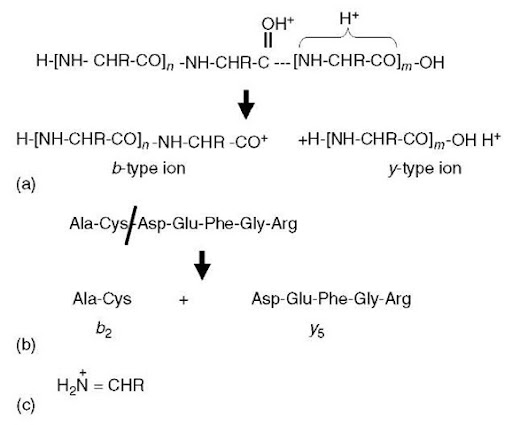Figure 1 Peptide fragment ions. (a) A doubly protonated peptide has one proton sequestered within the C-terminal portion of the molecule and the other is protonating the carbonyl oxygen. This latter protonation weakens the amide linkage (dotted line) to the point that the peptide ion fragments into a C-terminal y-type ion and an N-terminal b-type ion. (b) This example indicates that b- and y-type ions are numbered according to the number of residues each ion contains. (c) Structure of immonium ions

For the sake of simplicity, Figure 1a depicts b-type ions as acylium cations. However, it is currently thought that a more likely reaction mechanism involves nucleophilic attack of the protonated carbonyl carbon by the adjacent carbonyl oxygen located N-terminal to the cleavage site (Arnott et al., 1994; Schlosser and Lehmann, 2000). This would result in a five-member oxazolone ring structure on the C-terminal end of b-type ions. Such a fragmentation mechanism is appealing in that it accounts for certain well-known observations. First, one does not observe b1 ions (b-type ions comprised only of the N-terminal amino acid in the peptide) for peptides with free N-termini, since there is no carbonyl group to induce cleavage. In contrast, if a carbonyl is added to the N-terminal amino group, either by acylation or carbamylation, such modified peptides can fragment to produce b1 ions. Second, fragmentation on the C-terminal side of proline residues is much reduced, since the side chain ring structure constrains the attack of the carbonyl.

Table 1 Masses for monoisotopic amino acid residues (defined as —NH-CHR-CO-) calculated using all light isotopes. To calculate the mass of a y-type ion, sum the appropriate residue masses and add 19.0184 (three hydrogen plus one oxygen); to calculate the mass of a b-type ion, sum the appropriate residue masses and add 1.0078 (hydrogen atom)

 Amino acid Single-letter code Mass Glycine G 57.0215 Alanine A 71.0371 Serine S 87.0320 Proline P 97.0528 Valine V 99.0684 Threonine T 101.0477 Cysteine C 103.0092 Isoleucine I 113.0841 Leucine L 113.0841 Asparagine N 114.0429 Aspartic acid D 115.0270 Glutamine Q 128.0586 Lysine K 128.0950 Glutamic acid E 129.0426 Methionine M 131.0405 Histidine H 137.0589 Oxidized methionine 147.0354 Phenylalanine F 147.0684 Arginine R 156.1011 Carbamidomethylcysteine 160.0307 Tyrosine Y 163.0633 Tryptophan W 186.0793

A few ion types are observed that are not sequence specific. When a b-/y-type fragmentation (Figure 1a) occurs twice in the same molecule, one generates the so-called internal fragment ions. These possess neither the C- nor N-termini, and have a structure analogous to a b-type ion that is missing a piece of the N-terminus. These are particularly prominent when proline is on the N-terminal side of the internal fragment, and they rarely contain more than two to four amino acids. Immonium ions are composed of protonated single amino acid residues that have eliminated a carbon monoxide molecule (Figure 1c).

Shown in Figure 1a is the presence of a fragmentation-promoting proton located on the carbonyl oxygen. Although in acidic aqueous solutions amines and histidine imidazole rings are preferentially protonated over amide carbonyls, there is less of a difference in basicity in the gas phase. Once in the vacuum of a mass spectrometer, the protons on a peptide ion are relatively free to move about, and this “mobile proton theory” provides an important framework for understanding and predicting how peptides will fragment. An important exception seems to occur when the number of arginine residues exceeds the number of charging protons (Kapp et al., 2003). The guanido group of arginine has such high gas-phase basicity that it tends to sequester protons and makes them unavailable for inducing amide bond fragmentation. In the event that there is no amide protonation, higher collision energy is needed to mobilize the proton on the arginine. The higher collision energy can result in alternative and sometimes unexpected and incomplete fragmentation (e.g., Figure 2). The MS/MS spectrum of a doubly protonated peptide ion that contains only one arginine residue (i.e., there is one mobile proton) contains a contiguous series of y -type ions and is easily sequenced (Figure 2a). The spectrum of a peptide containing equal numbers of arginines and protons (no mobile proton) exhibits unusual fragment ions that do not delineate the entire sequence (Figure 2b). Electrospray-ionized tryptic peptides tend to produce easily sequenced MS/MS spectra (e.g., Figure 2a), since they typically have no more than one arginine and are usually multiply protonated (i.e., they have a mobile proton). Furthermore, by placing the arginine at the C-terminus, this helps ensure that a complete series of y-type ions are formed.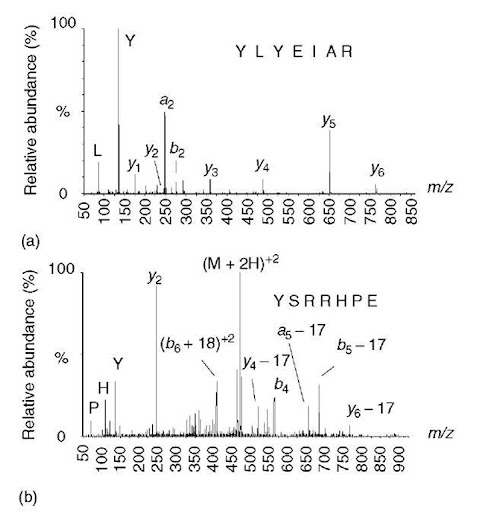Figure 2 Effect of a mobile proton on peptide fragmentation. (a) A doubly protonated peptide ion containing only one arginine leaves a single mobile proton available for promoting cleavages at amide bonds, as indicated in Figure 1(a). This spectrum exhibits a contiguous series of y-type ions that can be easily sequenced. (b) A doubly protonated peptide ion containing two arginine residues, leaving no mobile proton. In this spectrum, there are no contiguous series of ions from which the sequence can be deduced, but there are several unusual fragment ions – doubly charged b+18 ions, plus a preponderance of neutral losses from a few y-, b-, and a-type ions

The presence or absence of a mobile proton accounts for some well-known observations. First, cleavage on the C-terminal side of aspartic acid is enhanced for peptide ions lacking a mobile proton (Yu et al., 1993; Wysocki et al., 2000), which is thought to be due to self-protonation of the amide backbone by the acidic side chain. Second, cleavage on the N-terminal side of proline is enhanced for peptide ions containing a mobile proton (Wysocki et al., 2000). This is presumably due to skewing the population of amide protonation to favor N-alkylated amides, which have higher gas-phase basicity (Nair etal., 1996). Thus, the N-terminal side of proline is more likely to be protonated, and therefore suffer a cleavage.

## 3. General approach to sequencing peptides

If a single complete and contiguous series of a single ion type is present in a spectrum, then deducing the sequence is a simple matter of subtracting one fragment ion from the next (e.g., Figure 3). Each mass difference would correspond to one of the amino acid residue masses (Table 1). However, since it is not known if the ion series contains the C- or N-terminus (i.e., whether the series is due to y-type or b-type ions), the directionality of the derived sequence is not known. To determine this, one needs to be able to plausibly connect the series with one of the termini. For tryptic peptides, which have a C-terminal lysine or arginine, this can be done by determining if the lowest mass ion in the series corresponds to a y1 ion containing either lysine y = m/z 147) or arginine y = m/z 175) (Figure 3a). If the peptide is not derived from tryptic proteolysis, then one would look for y1 ions corresponding to any other possible amino acid (y = residue mass plus 19). Alternatively, one can check to see if the highest mass ion in the series is one residue short of accounting for the entire mass of the peptide (e.g., Figure 3b).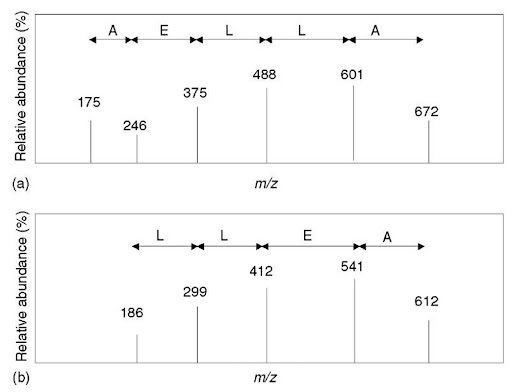Figure 3 General approach to peptide sequencing. Hypothetical spectra are shown of a peptide with a molecular weight of 785. (a) Fragment ion mass differences delineate a partial sequence; however, the directionality is not known (i.e., the partial sequence could be either AELLA or ALLEA). The lowest mass ion in the series at m/z 175 corresponds to a y 1 ion containing an arginine residue; therefore, a likely explanation is that the series contains the C-terminus -ALLEAR. This partial sequence is 114 Da short of the peptide mass (corresponding to N or two Gs), so two candidate sequences would be NALLEAR or GGALLEAR. (b) The fragment ion mass differences delineate two possible sequences (LLEA or AELL, directionality is unknown). The lowest mass ion in the series does not correspond to any possible y 1 ion (186 — 19 = 167, which does not correspond to an amino acid residue mass listed in Table 1). If the ion series was due to b-type ions, then the highest mass ion might be due to the loss of a single C-terminal residue. Subtract 17 Da from the peptide mass and then subtract the highest mass ion in the series (785 — 17 — 612 = 156). The residue mass of arginine is 156, so this could be assumed to be a b-type ion series. Hence, a partial sequence can be deduced as LLEAR, which is 185 Da less than the peptide mass. Therefore, there are two or more unsequenced amino acids with a combined mass of 185 Da that is appended to the N-terminus –  LLEAR

Interpreting MS/MS spectra is never as simple as what is depicted in Figure 3. Usually, there is more than one ion series, and, in addition, secondary fragmentations frequently occur (losses of water or ammonia). Internal fragment ions will cause confusion, and sometimes certain y- or b-type ions are missing. For example, it is very common to not observe fragmentation between the two N-terminal amino acids. In these cases, the exact sequence cannot be determined; one can only say that there are two or more amino acids with a certain combined mass that are located at the N-terminus. Additional ambiguity results from the fact that leucine and isoleucine have identical masses and cannot be distinguished. Glutamine and lysine differ by only 0.036 Da, and phenylalanine and oxidized methionine by 0.033 Da. These mass differences can be detected only with higher-mass-accuracy instruments that are well calibrated. Also, one needs to remember that certain pairs of amino acids have similar or identical masses as some single amino acids (G + G = N, A + G = Q, G + V ~ R, A + D ~ W, S + V ~ W). Some of these difficulties are demonstrated in the examples described below.

Different types of instruments will provide data with different characteristics. Two tryptic peptide examples will be shown below – one from an ion trap (Cooks and Kaiser, 1990) and the other from a quadrupole/time-of-flight (Qtof) hybrid (Morris et al., 1996). The ion trap that was used in this example exhibited lower resolution (unit resolved) and lower mass accuracy (+/- 0.4 Da); another characteristic of ion trap spectra is that part of the lower mass end of the MS/MS spectrum is absent. In contrast, the Qtof mass spectrometer provided full scans with sufficient resolution and accuracy to distinguish lysine from glutamine. Another difference is that ion trap spectra contain abundant high-mass b-type ions, whereas Qtof MS/MS spectra rarely exhibit b-type ions containing more than a few amino acids.

### 3.1. Example #1: ion trap spectrum

The approach taken in this example (Figure 4) is to first see if there are any partial sequences that can be easily determined from the least noisy region of the spectrum. For MS/MS spectra of multiply charged peptide ions, the least noisy region is located above the precursor m/z value (Figure 4b). In this region, there are two ion series (ions marked * or ~); the ion series marked by an asterisk also contains a series due to neutral loss of water. These ion series define two partial sequences – LSLV and LVES – however, the directionality is not known.

Directionality is surmised by trying to connect an ion series to one of the termini by assuming the series is either b-type or y-type (as described in Figure 3). Ion trap MS/MS spectra are missing the lower m/z end, so one cannot expect to find a y 1 ion to help identify the y-type series. On the other hand, high molecular weight b-type ions are frequently observed in ion trap spectra, so the next step is to see if the higher m/z ion from each series will correspond to the loss of arginine or lysine to form the highest mass b-type ion. In this example, the calculation is done by subtracting 17 (the mass of the C-terminal OH group) from the peptide molecular weight (1228, in this example), and then subtracting the highest m/z value from each of the two series (thus, 1228 — 17 — 987 = 224 and 1228 — 17 — 1083 = 128). The mass difference of 128 Da corresponds to the residue mass of lysine, and so the series marked by an asterisk can now be assumed to be a b-type ion series.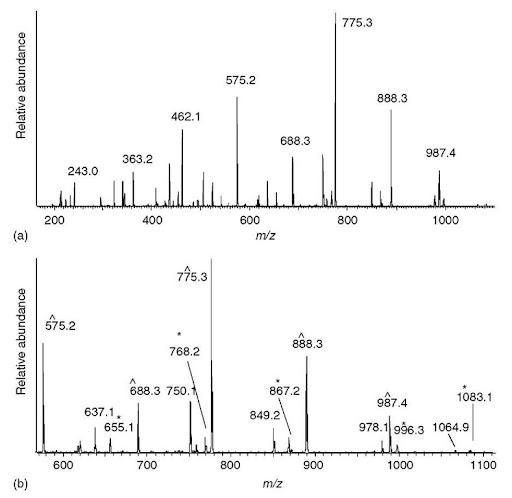Figure 4 Sequencing a peptide of molecular weight 1228.7 from an ion trap MS/MS spectrum of a doubly charged precursor at m/z 615.4. (a) Entire spectrum is shown with a few of the major peaks labeled. (b) The region above the precursor ion m/z usually contains the fewest ions and exhibits the simplest fragmentation pattern. One series of ions (labeled ~) have mass differences corresponding to the partial sequence LSLV. The other series of ions (labeled *) all have satellite peaks 18 Da lower and delineate a different partial sequence LVES. The ion at m/z 1083 has the correct mass for a b-type ion resulting from the loss of a single lysine residue; therefore, the ion series marked with an asterisk is hypothesized to be a b-type ion series that delineates the partial sequence LVESK. The other series is presumed to be a y-type series. (c) From the partial sequence LVESK, the low-mass y-type ions (y 1 to y5) can be calculated (see Table 1) — m/z 147, 234, 363, 462, and 575. The ions matching these calculated y-type ions are labeled (~), and are contiguous with the similarly labeled (presumed y-type ions) in panel (b). The low-mass b-type ions can be calculated from the high-mass y-type ions (see text) —m/z 243, 342, 455, 542, and 655 – which are contiguous with the similarly labeled ions in panel (b)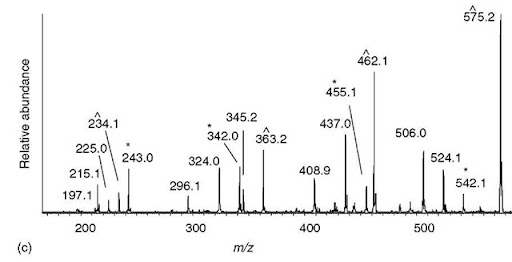Figure 4 (continued)

If no series can be identified as a b-type series, then it is sometimes possible to see if the mass difference obtained could correspond to lysine or arginine plus another amino acid. For example, if m/z 1083 was absent and only m/z 996 was visible (the next ion in the series below 1083), then the mass difference would be 215. Since no combination of lysine or arginine plus another amino acid can equal 224, and since serine plus lysine equals 215, one could still tentatively hypothesize that the asterisk-labeled series is due to b-type ions. In any case, two partial sequences are known at this point – the sequence LVESK (derived from the b-type ion series), plus VLSL located toward the N-terminal part of the sequence (which by the process of elimination is now assumed to be a y-type ion series).

The next step is to connect these two sequences and possibly extend them. From the partial sequence LVESK, the low-m/z y-type ions can be calculated – add 19 to the residue mass of lysine to obtain y 1, add the residue mass of serine to the y 1 mass to get y2, and so on (Figure 4c). The mass of y1 is usually below the mass range of an ion trap MS/MS spectrum, and one would not expect to see it. The other y-type ions (y2 through y5) are all present. The y5 ion happens to be the lowest m/z ion in the series that defined the partial sequence VLSL (Figure 4b), which indicates that the two partial sequences are linked (VLSLLVESK). Similarly, low-mass b-type ions can be calculated from the high-m/z y-type ions by adding the mass of two protons to the peptide molecular weight and then subtracting the y-type ion mass (i.e., b-type ions = peptide MW + 2 hydrogen atoms – y-type ion). For example, the lowest mass b-type ion is calculated from the highest mass y-type ion (b-type ion = 1228 + 2 – 987 = 243). This b-type ion is composed of more than one amino acid, most likely two; hence, one could tentatively assign the ion at m/z 243 to be b2. The b3 through b6 can be calculated in a similar fashion, all of which are found in the spectrum. The b6 ion matches the lowest mass b-type ion in the series that defined the partial sequence of LVESK, which helps confirm that the two partial sequences are linked (VLSLLVESK). The only missing piece is at the N-terminal region where there is what is presumed to be a dipeptide of mass 242 Da. This region cannot be sequenced, since there is no b1 ion or y 10 ion present.

The sequence, as best can be defined by low-energy CID, is VLSLLVESK, where the number in brackets represents the combined mass of two or more amino acids. Also, the notation “L” implies either leucine or isoleucine, since the two cannot be distinguished.

At this point, one should verify that the majority of fragment ions can be accounted for, given this hypothetical sequence. This is accomplished by checking to see if there are any doubly charged b- or y -type ions, which is done by adding the mass of a proton to the singly charged b- or y-type ion and dividing the sum by two. It is unusual to see multiply charged fragment ions unless the precursor ion had more than two charges, although higher-mass doubly charged y-type ions are sometimes observed from doubly charged precursor ion. Internal fragments (fragments not containing either the C- or N-terminal amino acids) with 2 to 5 residues are sometimes seen, and these m/z values are calculated by summing the amino acid residue masses and then adding a proton. Finally, and most likely, are ions resulting from neutral losses of water and ammonia from the b – and y -type ions. Given these additional fragment ions, plus the y- and b-type fragment ions, all of the major ions can be accounted for, and in fact, the correct sequence is ELVISLIVESK. Losses of water from b-type ions are particularly prominent in this example (Figure 4), which is due to the presence of an N-terminal glutamic acid that readily formed an N-terminal pyroglutamyl N-terminus during the fragmentation process. Similarly, prominent losses of ammonia are seen for peptides with glutamine at the N-terminus.

### 3.2. Example #2: Qtof spectrum

Most tryptic peptides have lysine or arginine at their C-terminus, and the approach taken in this example (Figure 5) is to first see if there are any y1 ions containing lysine or arginine (m/z 147.113 and 175.119, respectively). The aim is to build the sequence toward the N-terminus in a stepwise fashion. In this approach, the directionality of the sequence is not an issue, since one starts out with what is presumed to be a C-terminal y 1 ion. In this spectrum (Figure 5a), an ion at m/z 147.111 is observed, and therefore a C-terminal lysine is presumed to be present. The mass of the y1 ion is subtracted from higher-m/z fragment ions in a search for mass differences corresponding to an amino acid residue mass, and two such ions are found at m/z 262.141 (y 1 plus aspartic acid) and 333.177 (y 1 plus tryptophan). Since tryptophan has about the same mass as aspartic acid plus alanine, one could potentially account for both ions by assuming that the penultimate residue is Asp and that the y2 ion is m/z 262.141. Using the same approach to find y3 ions, m/z 262.141 is subtracted from higher-m/z fragment ions, and two ions are found at m/z 333.177 and 409.283, corresponding to alanine and phenylalanine. Whereas the mass difference between m/z 333.177 and 262.141 is almost exactly the correct mass for alanine (Table 1), the mass difference between m/z 409.283 and 262.141 is in error by 0.074 Da compared to the correct mass for phenylalanine. For Qtof data, this error is too much, and the y3 ion is probably m/z 333.177. At this point, likely y1 — y3 ions have been identified that define a partial sequence at the C-terminal end of the peptide (ADK). Continuing this process, two potential y4 ions are identified at m/z 462.220 and 489.251, corresponding to additions of glutamic acid and arginine respectively. The errors in mass differences between these two ions and the y3 ion at m/z 333.177 are both sufficiently small that it is not possible to choose between the two. Hence, further sequence extensions have to proceed, keeping both possibilities in mind. However, there are no ions above m/z 489.251 whose mass difference corresponds to a single residue. In contrast, the ion at m/z 577.249 is almost exactly the mass of aspartic acid above m/z 462.220. The remainder of the spectrum contains a series of ions from m/z 664.281 to 1076.549 whose mass differences delineate a partial sequence of SLSLV.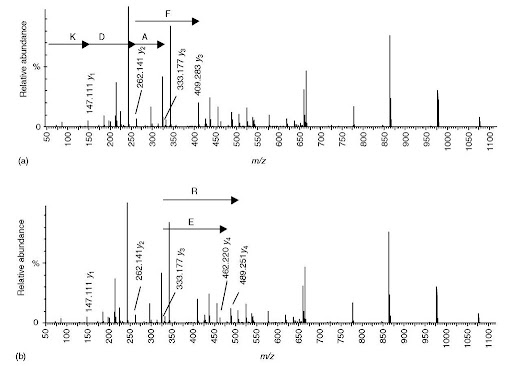Figure 5 Sequencing a peptide of molecular weight 1317.67 from a Qtof MS/MS spectrum of a doubly charged precursor at m/z 659.8. (a) The _vi ion representing a C-terminal lysine is present at m/z 147.111. There are two ions at m/z 262.141 and 333.177 that differ in mass from the _vi ion by an amino acid residue mass (aspartic acid and tryptophan, respectively). Since the residue mass of tryptophan is approximately the same as the combined mass of aspartic acid and alanine, an additional ion is accounted for by assuming that the C-terminal sequence is ADK (_V2 = m/z 262 and y3 = m/z 333.177) rather than WK (V2 = 333.177). The mass accuracy of the Qtof instrument excludes an alternative y3 ion at m/z 409.283. (b) There are two potential 74 ions; however, for m/z 489.251. there are no other ions at higher m/z that differ by an amino acid residue mass (i.e.. it is a dead end), (c) The putative 74 ion at m/z 462.220 is the low-mass end of a series of ions that unambiguously define a partial sequence containing six amino acids, (d) Most of the remaining ions not hypothesized to be v-type ions (as described in panels a-c) are identified as a- or f>-type ions, plus some neutral losses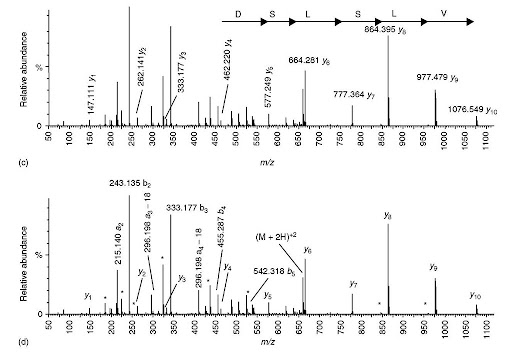Figure 5 (continued)

From the highest-mass y-type ion at m/z 1076.549, one can calculate a low-mass b-type ion at m/z 243.14 (b-type ions = peptide MW + 2 hydrogen atoms — y-type ion). This b-type ion is composed of more than one residue, most likely two. As in Example #1, the next step is to verify that the remaining abundant ions can be accounted for as either b- or y-type ions, multiply charged ions, internal fragment ions, neutral losses, or immonium ions. Given the limitations of low-energy CID, one can rationally determine the sequence to be VLSLSDEADK, where 242 implies the presence of more than one residue whose residue mass sum is 242. Likewise, L signifies either isoleucine or leucine; however, using the answer to Example #1 as a clue, the reader may be able to correctly guess the entire sequence.

## 4. Conclusion

Deriving sequences from tandem mass spectra is slow and tedious, but not particularly difficult. Most people have acquired the necessary mathematics skills (addition and subtraction) by the age of eight, although the possibility of multiple charging resulting from electrospray ionization may require more advanced math skills (multiplication and division). The main impediment for most people is that there is not enough time to interpret all the spectra generated. For this reason, there have been a number of attempts to automate the process using various computer programs and algorithms (e.g., Bartels, 1990; Biemann et al., 1966; Chen et al., 2001; Dancik et al., 1999; Fernandez-de-Cossio et al., 2000; Ma, 2003); although this is an important topic, further discussion of these programs is beyond the scope of this tutorial.

Next post:

Previous post: